# 5 5 Polynomials 1 2 3 Goals To

• Slides: 15
Download presentation5. 5 Polynomials • 1. 2. 3. Goals: To identify a polynomial and its types To identify terms, coefficients To identify the degree of the poly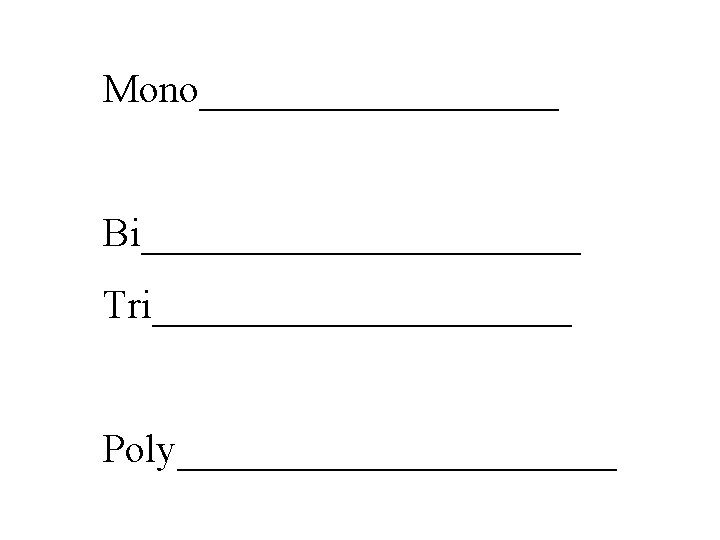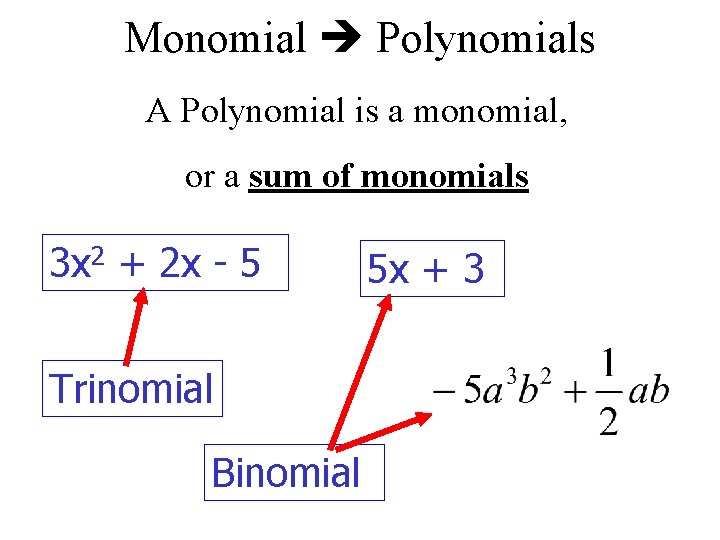Monomial Polynomials A Polynomial is a monomial, or a sum of monomials 3 x 2 + 2 x - 5 Trinomial Binomial 5 x + 3Review: What is a monomial? • • • 1 term Number Variable Number & Variable Multiplied Exponent (as long as the exponent is a whole number, no fraction, no decimal and no negative exponents allowed for a mono) • No variables in the denominator • No + or - signs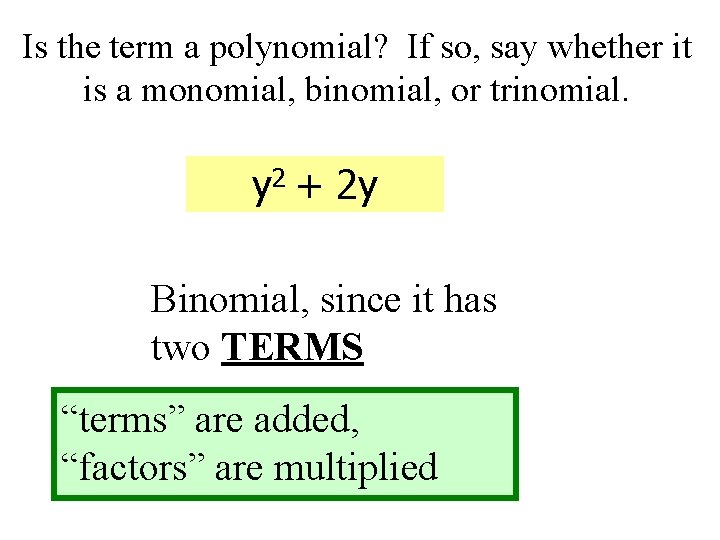Is the term a polynomial? If so, say whether it is a monomial, binomial, or trinomial. y 2 + 2 y Binomial, since it has two TERMS “terms” are added, “factors” are multipliedIs the term a polynomial? If so, say whether it is a monomial, binomial, or trinomial. No “terms” are added, “factors” are multipliedIs the term a polynomial? If so, say whether it is a monomial, binomial, or trinomial. YES, trinomial since it has 3 terms Numeric factor of a term is called the coefficient.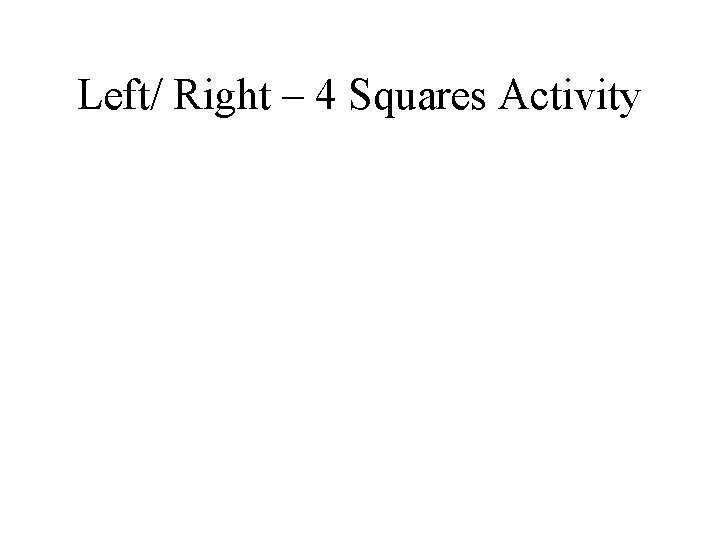Left/ Right – 4 Squares Activity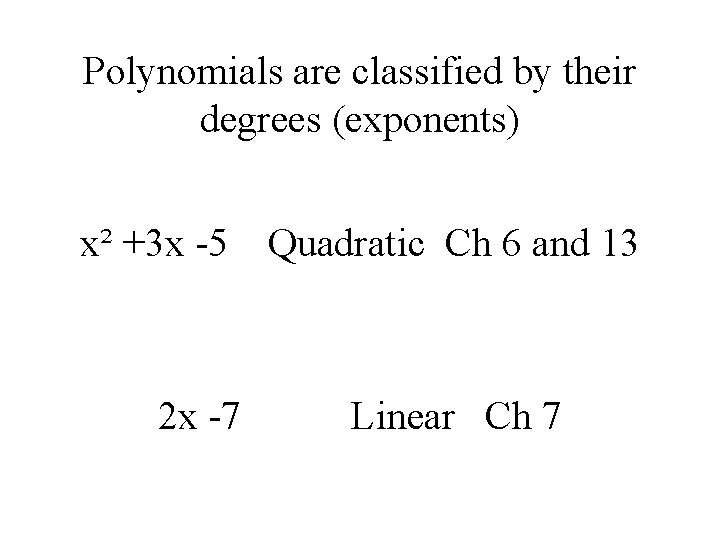Polynomials are classified by their degrees (exponents) x² +3 x -5 2 x -7 Quadratic Ch 6 and 13 Linear Ch 7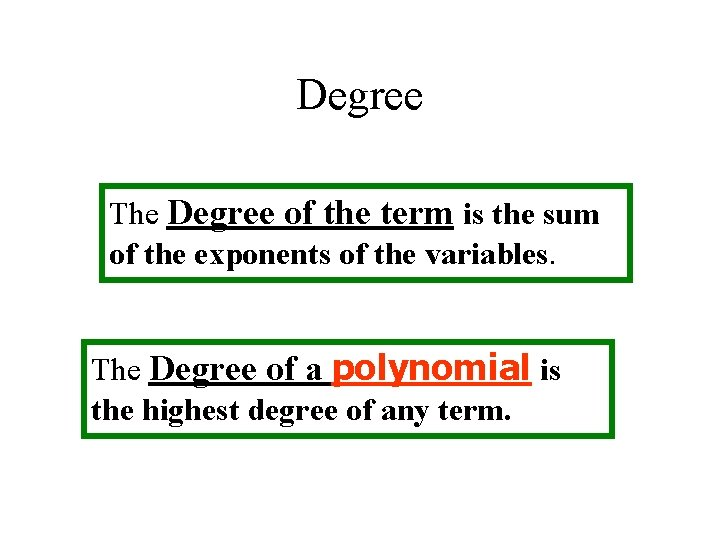Degree The Degree of the term is the sum of the exponents of the variables. The Degree of a polynomial is the highest degree of any term.Identify the degree of each term and the degree of the polynomial. - 63 x 2 y 3 – 16 xy 5 + y 4 5 th deg 6 th deg 4 th deg This is a 6 th degree polynomial. The Degree of a term is the sum of the exponents of the variables. The Degree of a polynomial is the highest degree of any term.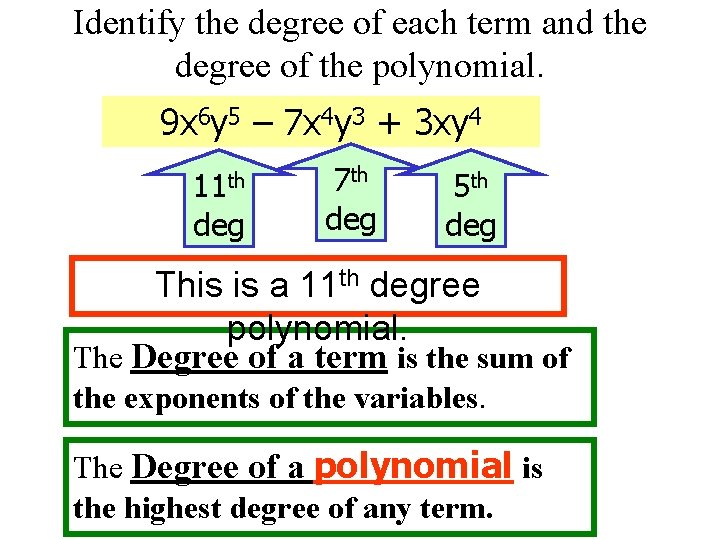Identify the degree of each term and the degree of the polynomial. 9 x 6 y 5 – 7 x 4 y 3 + 3 xy 4 11 th deg 7 th deg 5 th deg This is a 11 th degree polynomial. The Degree of a term is the sum of the exponents of the variables. The Degree of a polynomial is the highest degree of any term.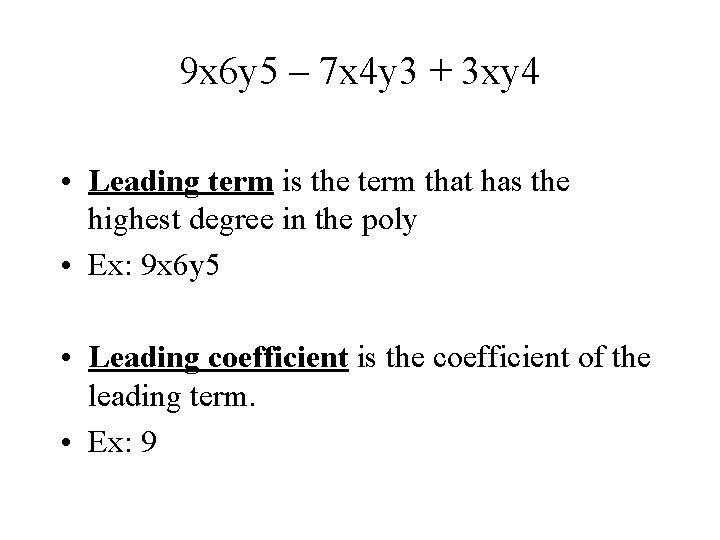9 x 6 y 5 – 7 x 4 y 3 + 3 xy 4 • Leading term is the term that has the highest degree in the poly • Ex: 9 x 6 y 5 • Leading coefficient is the coefficient of the leading term. • Ex: 9Hold up the degree of the polynomial with your appropriate fingersAssignment: Page 224 (2 -24, 42 -46) even